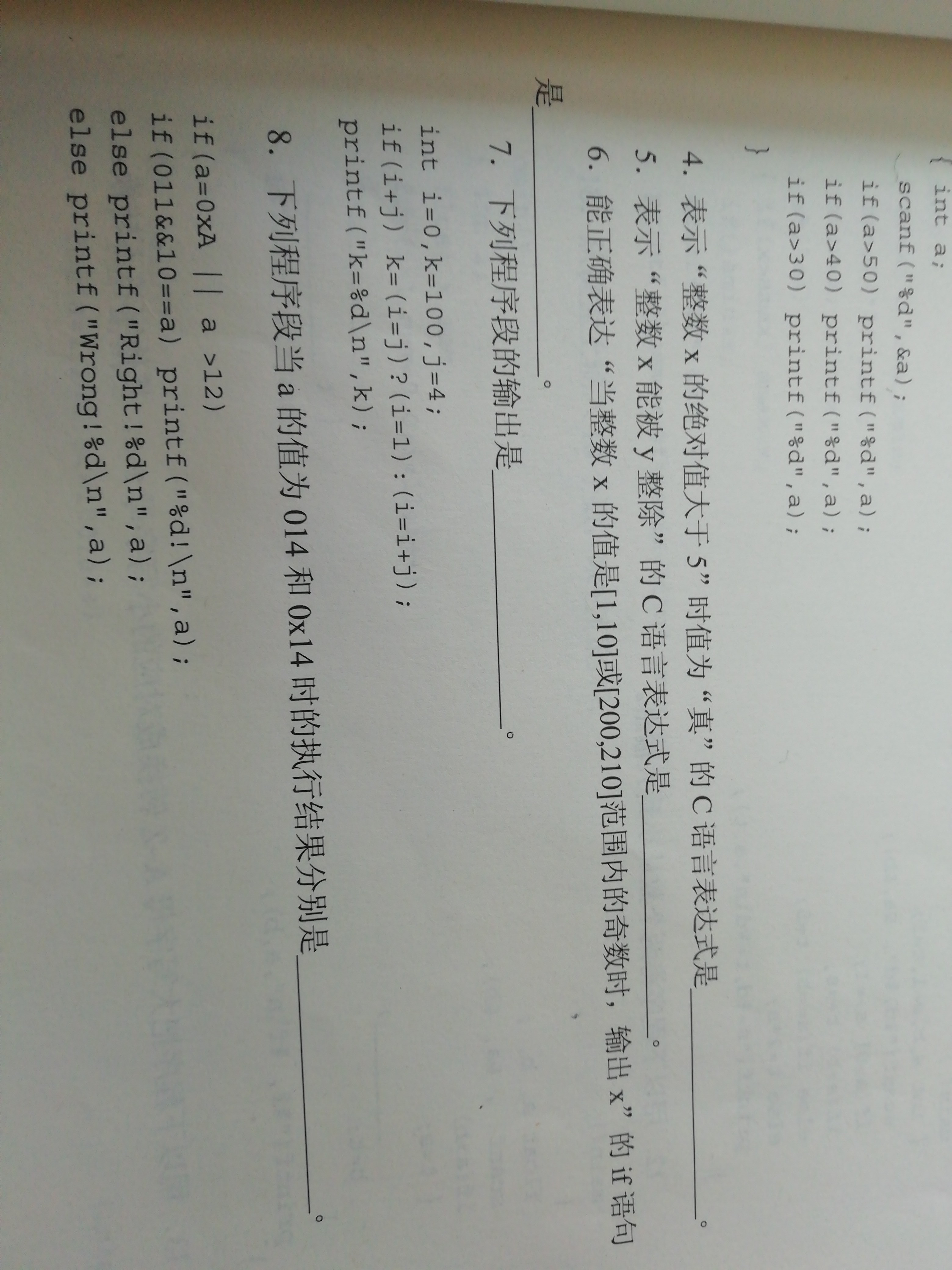###### Eivae

2020-02-24 09:56 阅读 323

# 请问第七题为什么不是输出k=4？k=(i=j)?(i=1):(i=i+j);这里，把j赋给了i，所以i就等于4,再把i=4赋给了k，所以k=4为非零，所以执行i=1，这样i就等于了1。所以最后是k=4而i=1。这样分析为什么不正确？（C语言小白）

• 点赞
• 写回答
• 关注问题
• 收藏
• 复制链接分享

#### 2条回答默认 最新

• 已采纳

if (i+j)这个true
(i=j=4)所以为真
所以k=i=1，也就是k=1

点赞 1 评论 复制链接分享
• ``````k=(i=j)?(i=1):(i=i+j)
``````

注意，是`i=j`不是`i==j`,是成立的。所以执行k=i=1,k就是1

点赞 1 评论 复制链接分享